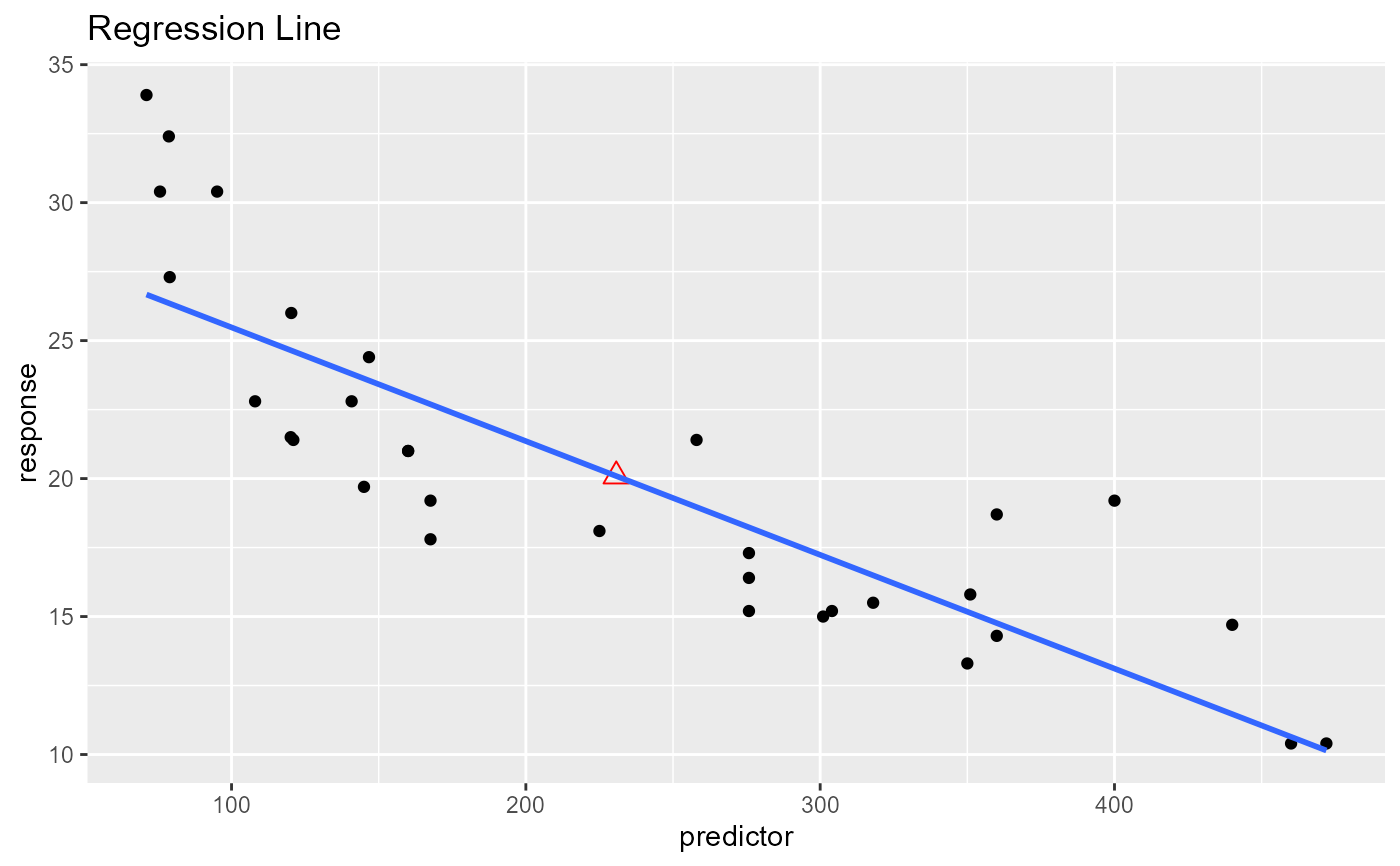Plot to demonstrate that the regression line always passes through mean of the response and predictor variables.

## Usage

ols_plot_reg_line(response, predictor, print_plot = TRUE)

## Arguments

response

Response variable.

predictor

Predictor variable.

print_plot

logical; if TRUE, prints the plot else returns a plot object.

## Examples

ols_plot_reg_line(mtcars$mpg, mtcars$disp)
#> geom_smooth() using formula = 'y ~ x'